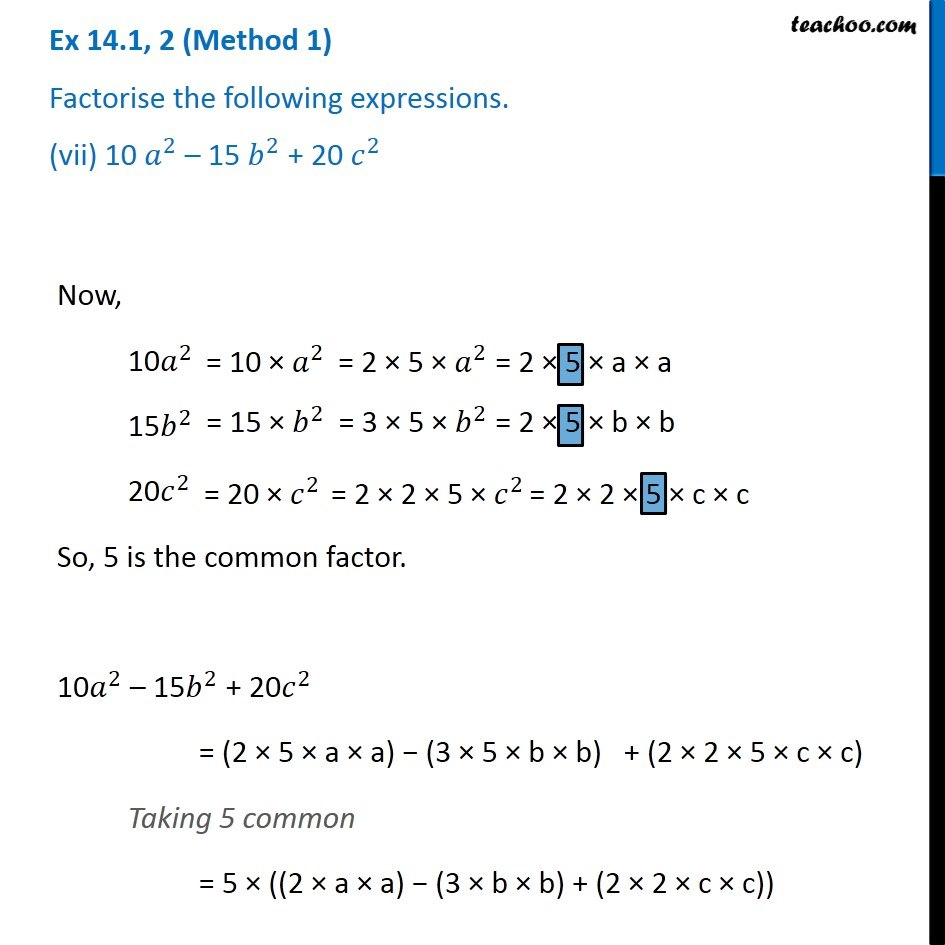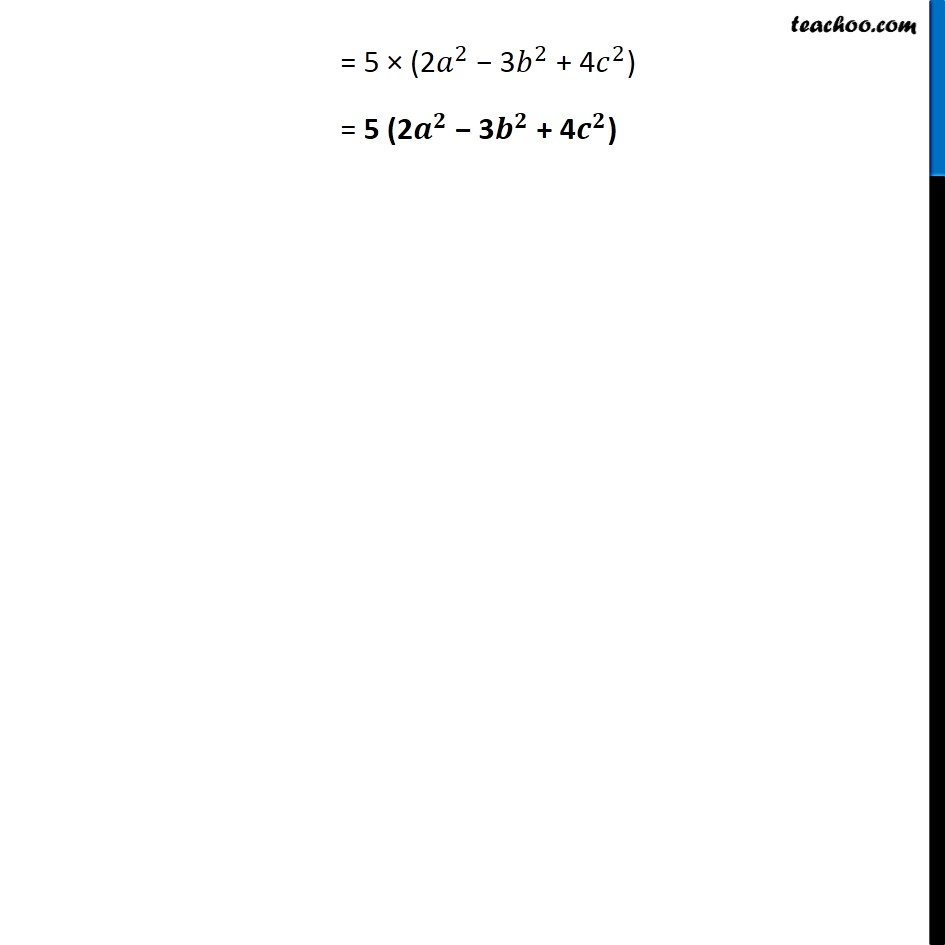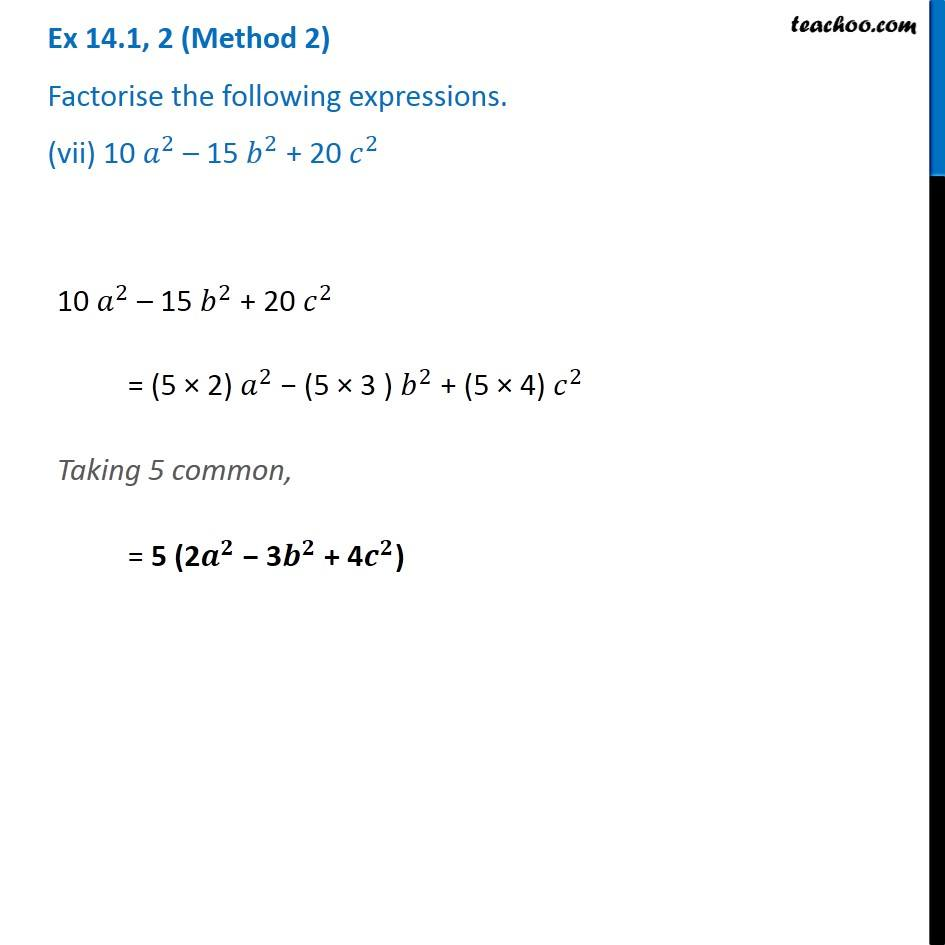Factorisation using common factors

Chapter 12 Class 8 Factorisation
Concept wiseLearn in your speed, with individual attention - Teachoo Maths 1-on-1 Class

### Transcript

Ex 12.1, 2 (Method 1) Factorise the following expressions. (vii) 10 𝑎^2 – 15 𝑏^2 + 20 𝑐^2 Now, 10𝑎^2= 10 × 𝑎^2 = 2 × 5 × 𝑎^2 = 2 × 5 × a × a 15𝑏^2 = 15 × 𝑏^2 = 3 × 5 × 𝑏^2 = 2 × 5 × b × b 20𝑐^2 = 20 × 𝑐^2 = 2 × 2 × 5 × 𝑐^2 = 2 × 2 × 5 × c × c So, 5 is the common factor. 10𝑎^2 – 15𝑏^2 + 20𝑐^2 = (2 × 5 × a × a) − (3 × 5 × b × b) + (2 × 2 × 5 × c × c) Taking 5 common = 5 × ((2 × a × a) − (3 × b × b) + (2 × 2 × c × c)) = 5 × (2𝑎^2 − 3𝑏^2 + 4𝑐^2) = 5 (2𝒂^𝟐 − 3𝒃^𝟐 + 4𝒄^𝟐) Ex 12.1, 2 (Method 2) Factorise the following expressions. (vii) 10 𝑎^2 – 15 𝑏^2 + 20 𝑐^2 10 𝑎^2 – 15 𝑏^2 + 20 𝑐^2 = (5 × 2) 𝑎^2 − (5 × 3 ) 𝑏^2 + (5 × 4) 𝑐^2 Taking 5 common, = 5 (2𝒂^𝟐 − 3𝒃^𝟐 + 4𝒄^𝟐)